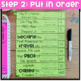# 3rd Grade Math Review | End of Year Math Puzzles | Cut and Paste OptionSubject
Resource Type
Format
PDF (143 MB|125 pages)
\$5.00
Also included in
1. Are you a SECOND GRADE teacher? Here's the 2nd Grade bundle.©Unravel the Fact Math Puzzles are an original idea by The Teacher Wears Prada. Click here to read a blog post about these Unravel the Fact Math PuzzlesHow do these low-prep math puzzles work? Each monthly unit of these cross-curricular Unr
\$30.00
\$41.00
Save \$11.00

#### Product Description

Are you looking for the 2nd Grade End of the Year Math Puzzles?

©Unlock the Fact Math Puzzles are an original idea by The Teacher Wears Prada.

>>>>CLICK HERE to read a blog post about these Unlock the Fact Math Puzzles and see different examples of this resource, how it works, etc.

These math puzzles are perfect for end of the year math review and keeping your students engaged while their minds are thinking about all things summer!

How do these low-prep math puzzles work?

The MAY/End of the Year EDITION of these cross-curricular Unlock the Fact Math Puzzles reveal facts themed around holidays in May. Students will answer the 9 math problems, put the puzzle strips in order from LEAST to GREATEST. If they have answered the problems correctly and have placed the numbers in order correctly, an interesting fact will be revealed. They will have "unlocked a fact".

Content Integration + Self-Correcting Math Puzzles = Happy Teacher and Engaged Students

What themes/facts are featured this month?

Asian Pacific American Heritage Month, National Space Day (facts about Planets), National Astronaut Day (facts about Astronauts), National Tuna Day (facts about tuna), National Shrimp Day (facts about shrimp), National Bike Day (facts about history of bikes), National Train Day (facts about history of trains), Cinco de Mayo, Memorial Day, Kentucky Derby, National Egg Month, National Love a Tree Day (facts about trees)

This resource includes 50 different math puzzles {two different formats} that cover a variety of 3rd Grade Common Core Math Skills. A recording sheet for accountability and a fact booklet for students to record their facts {optional} are also included.

Two different formats included:

Option #1: {MATH CENTERS} Print on colored paper, laminate, students write and wipe the answers with a thin dry erase marker. These laminated puzzles can be placed in math centers.

OR

Option #2: {PRINT AND GO RESOURCE} Pieces are already mixed up/jumbled up for students to solve, cut and put together to glue into math notebook or on a piece of construction paper. Students can complete these independently for morning work, math warm-ups, independent math work during math rotations, etc.

What skills are included?

{The Common Core standard is printed in the upper left corner of each puzzle}

• Multistep Word Problems
• 3 Digit Subtraction and Addition Word Problems
• Multiplication Word Problems
• Division Word Problems
• Measurement Word Problems
• Telling time to the minute
• Elapsed Time
• Counting Money (up to \$5)
• Identifying Fractions
• Comparing Fractions
• Fractions on a Number Line
• Fractions Word Problems
• Equivalent Fractions
• Improper Fractions
• Adding and Subtracting Fractions (Same Denominator)
• Mixed Three Digit Addition and Subtraction with regrouping
• Graphing (pictograph, line plot)
• Graphing (bar graphs)
• Perimeter of Polygons
• Perimeter of Polygons (Find Missing Length)
• Perimeter Word Problems
• Perimeter (Square Tiles)
• Area (Square Tiles)
• Area of Rectangles
• Area of Rectangles (Missing Sides)
• Area Word Problems
• Estimating Weight
• Estimating Capacity
• Identifying Polygons
• Multiplication (Two by One Multiplication)
• Multiplication (groups of)
• Multiplication (missing factors)
• Multiplication (arrays)
• Multiplication Facts
• Distributive Property
• Division Facts
• Mixed Multiplication and Division Facts
• Input and Output Pattern Tables (Multiplication)
• Rounding
• Place Value
• 4 Digit Addition and Subtraction
• 3 Digit Addition and Subtraction
• Missing Number (3 digit addition/subtraction)

___________________________________________________

Be the first to know about my new discounts, freebies and product launches:

If you're interested in more free resources, you can get access to my Free Resource Library here.

Connect with me:

Total Pages
125 pages
N/A
Teaching Duration
N/A
Report this Resource to TpT
Reported resources will be reviewed by our team. Report this resource to let us know if this resource violates TpT’s content guidelines.
• Ratings & Reviews
• Q & A

Teachers Pay Teachers is an online marketplace where teachers buy and sell original educational materials.